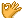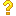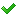•闪存
•博客
• 发言小组
• 投递新闻
• 提问博问
• 添加收藏
• 发布招聘
•文库

1

##返回指针值的函数

#include <stdio.h> int main(){ float score[]={ {60, 70, 80, 90},{56, 89, 67, 88},{34, 78, 90, 66}
6

4

2

##用c语言编写一个分子分母的最简式

#include<stdio.h>int main(){ int a,b,c,*d=0,*e=0; scanf("%d/%d",&a,&b); if(b==0)printf("no"); else i
1

##20查找c++程序 语法错误 有个程序

1 #include<iostream> 2 #include<algorithm> 3 using namespace std; 4 class Re_public_area 5 { 6 priva
5

##10char **p的用法

1 void t(char **p2){ 2 char *p1 ; 3 p1 = (char *)malloc(1); 4 *p2 = p1; 5 } 6 int main(){ 7 char **p
1

2

4

3

3

1

2

4

2

5

2

##json 访问action 返回数据在前端显示

1 \$(".sortCondition").click(function(){ 2 var condition=\$(this).text(); 3 alert(condition); 4 \$.ajax
1

##5关于数据在内存中的储存

#include<bits/stdc++.h>using namespace std;int main(){ int a=10; char b='c'; float c=10.0; double d=
3

5

2

2

##100jqajxa el获取session为空

<body>html <c:if test="\${not empty uname }">欢迎你:\${uname}</c:if> <c:if test="\${empty uname }"> <div i
2

3

##这两行代码各是什么意思？新手上路，请多指教

var data = new { msg = "a", result = false }; result = new { data = "b", msg = false };
2